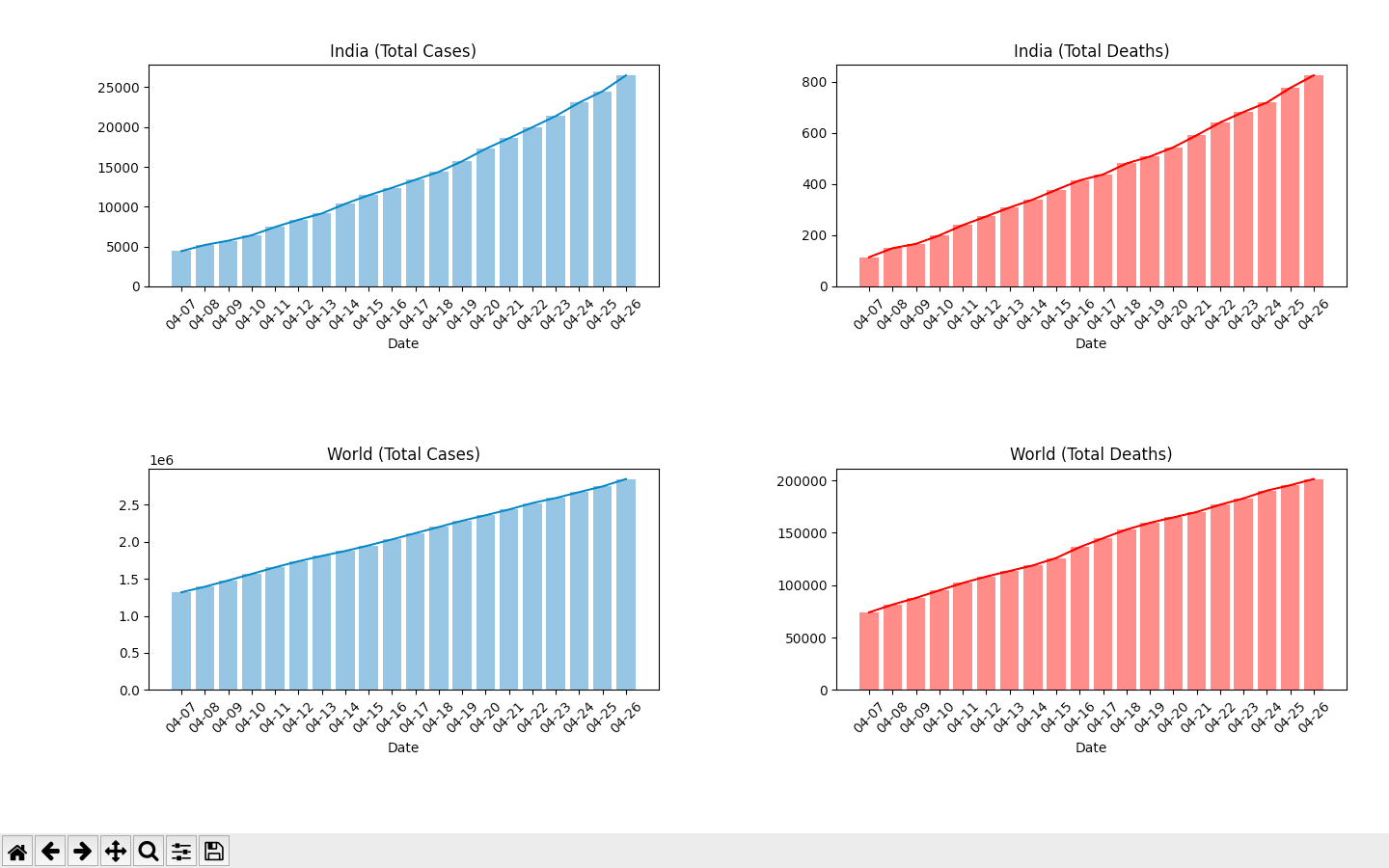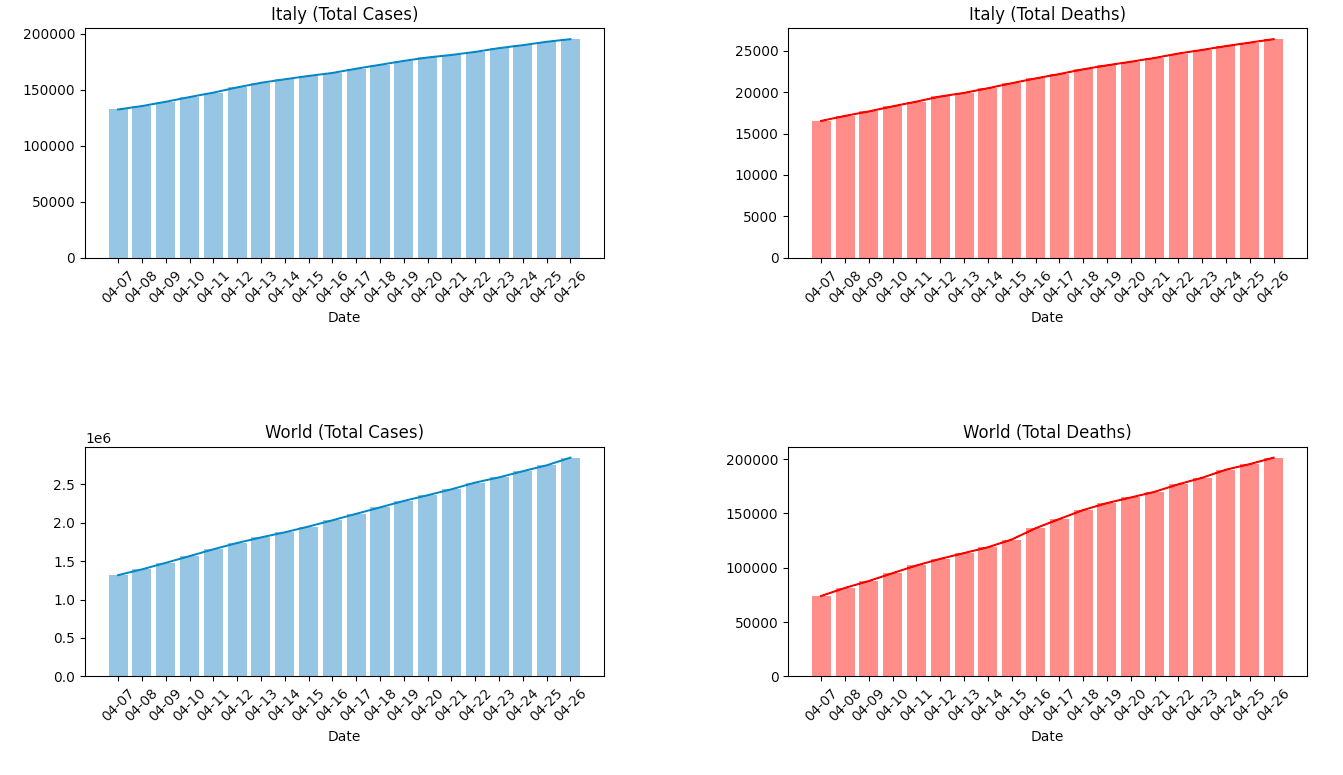# Plotting the Growth Curve of Coronavirus in various Countries using Python

• Last Updated : 29 Dec, 2020

In this article, we will collect latest updated information about the coronavirus cases across the world and in a particular country. We will plot graphs to visualise the growth of total number of cases and the total deaths for the last 20 days. The latest data is fetched from Our World in Data.

### Python modules required

• requests:
The requests library is used for making HTTP requests in Python.

Attention geek! Strengthen your foundations with the Python Programming Foundation Course and learn the basics.

To begin with, your interview preparations Enhance your Data Structures concepts with the Python DS Course. And to begin with your Machine Learning Journey, join the Machine Learning - Basic Level Course

```pip install requests
```
• matplotlib:
matplotlib is a comprehensive library for creating various types of graphs and interactive visualisations in Python.

```pip install matplotlib
```

Explanation:

The data scraped from the website(using requests), is converted to the csv-like format. Then this data is filtered to get the required statistics for the last 20 days and the visualizations are plotted(using matplotlib).

Below is the implementation.

 `from` `matplotlib ``import` `pyplot as plt``import` `requests``  ` ` ` `# function to plot data for country ``# and world``def` `Plot(country):``  ` `    ``# getting request from the url``    ``req ``=` `requests.get(``'https://covid.ourworldindata.org / data / ecdc / full_data.csv'``)``    ``req.raise_for_status()``      ` `    ``# converting to text and splitting ``    ``# the rows of the csv data``    ``cf ``=` `req.text.split(``'\n'``)``  ` `    ``# converting to 2 dimensional list``    ``for` `i ``in` `range``(``len``(cf)):``        ``cf[i]``=` `cf[i].split(``', '``)  ``      ` `      ` `    ``dates ``=``[]``    ``total ``=``[]``    ``total_w ``=``[]``    ``deaths ``=``[]``    ``deaths_w ``=``[]``    ``l ``=``[]``    ``f ``=` `0``  ` `      ` `    ``for` `i ``in` `range``(``len``(cf)``-``1``):``        ``l ``=` `cf[i]``        ``c ``=` `l[``1``]``         ` `        ``# filtering data for a particular country``        ``if` `c ``=``=` `country:``            ``f ``=` `1``             ` `            ``# getting the dates, total cases and``            ``# deaths for the particular country``            ``dates.append(l[``0``][``5``:])      ``            ``total.append(``int``(l[``4``]))``            ``deaths.append(``int``(l[``5``]))``              ` `                 ` `        ``# filtering data for the world``        ``if` `c ``=``=``'World'``:``  ` `            ``# getting total cases and deaths for``            ``# the world``            ``total_w.append(``int``(l[``4``]))``            ``deaths_w.append(``int``(l[``5``]))``              ` `    ``if` `f ``=``=` `0``:``        ``print``(``"Invalid country name."``)``        ``return``  ` `       ` `    ``# Plotting country data``    ``total_ax ``=` `plt.subplot(``2``, ``2``, ``1``)``    ``total_ax.set_title(country``+``' (Total Cases)'``)``      ` `    ``# plotting the curve for total cases``    ``total_ax.plot(dates[``-``20``:], total[``-``20``:])``      ` `    ``# plotting the bars for total cases``    ``total_ax.bar(dates[``-``20``:], total[``-``20``:], alpha ``=` `0.5``)``      ` `    ``total_ax.set_xlabel(``"Date"``)``    ``plt.xticks(rotation ``=` `45``)``  ` `  ` `  ` `  ` `  ` `    ``death_ax ``=` `plt.subplot(``2``, ``2``, ``2``)``    ``death_ax.set_title(country``+``' (Total Deaths)'``)``  ` `    ``# plotting the curve for deaths``    ``death_ax.plot(dates[``-``20``:], deaths[``-``20``:], color ``=``'red'``)``      ` `    ``# plotting the bars for deaths``    ``death_ax.bar(dates[``-``20``:], deaths[``-``20``:], color ``=``'red'``, alpha ``=` `0.5``)``      ` `    ``death_ax.set_xlabel(``"Date"``)``    ``plt.xticks(rotation ``=` `45``)``  ` `  ` `   ` `    ``# Plotting world data``    ``total_w_ax ``=` `plt.subplot(``2``, ``2``, ``3``)``    ``total_w_ax.set_title(``'World (Total Cases)'``)``  ` `    ``# plotting the curve for total cases``    ``total_w_ax.plot(dates[``-``20``:], total_w[``-``20``:])``  ` `    ``# plotting the bar for total cases``    ``total_w_ax.bar(dates[``-``20``:], total_w[``-``20``:], alpha ``=` `0.5``)``  ` `    ``total_w_ax.set_xlabel(``"Date"``)``    ``plt.xticks(rotation ``=` `45``)``  ` `  ` `  ` `      ` `      ` `    ``death_w_ax ``=` `plt.subplot(``2``, ``2``, ``4``)``    ``death_w_ax.set_title(``'World (Total Deaths)'``)``  ` `    ``# plotting the curve for deaths``    ``death_w_ax.plot(dates[``-``20``:], deaths_w[``-``20``:], color ``=``'red'``)``  ` `    ``# plotting the curve for deaths``    ``death_w_ax.bar(dates[``-``20``:], deaths_w[``-``20``:], color ``=``'red'``, alpha ``=` `0.5``)``  ` `    ``death_w_ax.set_xlabel(``"Date"``)``    ``plt.xticks(rotation ``=` `45``)``      ` `  ` `    ``plt.tight_layout()``        ` ` ` `print``(``"Enter country name..."``)``country ``=` `input``().title()``Plot(country)``plt.show()`

Input :

```Enter country name...
India```

Output :Input :

```Enter country name...
Italy```

Output :My Personal Notes arrow_drop_up